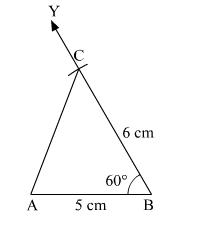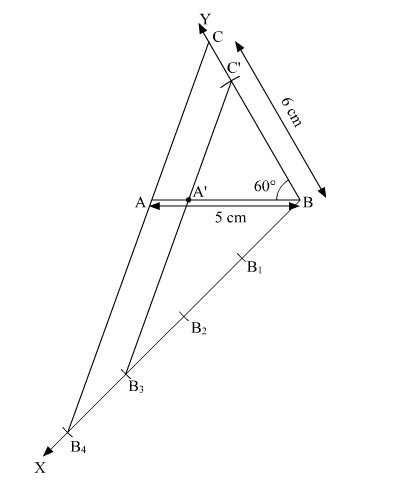# Construct a ΔABC with BC = 6 cm, ∠B = 60° and AB = 5 cm.`
Question:

Construct a $\triangle A B C$ with $B C=6 \mathrm{~cm}, \angle B=60^{\circ}$ and $A B=5 \mathrm{~cm}$. Construct another triangle whose sides are $\frac{3}{4}$ times the corresponding sides of $\triangle A B C$.

Solution:

Given: In ΔABC,
BC = 6 cm,
B  = 60°
AB = 5 cm

Steps of construction:
(1) Draw a line segment AB = 5 cm.
(2) From the point B, draw an ∠ABY = 60°
(3) Taking B as center, 6 cm radius, draw an arc on the ray BY,
Let the point where the arc intersects the ray named as C.
(4) Join AC.Hence,  ΔABC is the required triangle.

Now, we construct another triangle whose sides are $\frac{3}{4}$ times the corresponding sides of $\triangle A B C$.

Steps of construction:
(1) Draw any ray BX making an acute angle with BA on the side opposite to the vertex C.
(2) Mark four points B1B2Band B4 on BX, so that BB1 = B1B2 = B2B3 = B3B4.
(3) Join B4A and draw a line through Bparallel to B4to intersect AB at A'.
(4) Draw a line through A' parallel to AC to intersect BC at C'.Hence,  Δ​A'BC' is the required triangle.